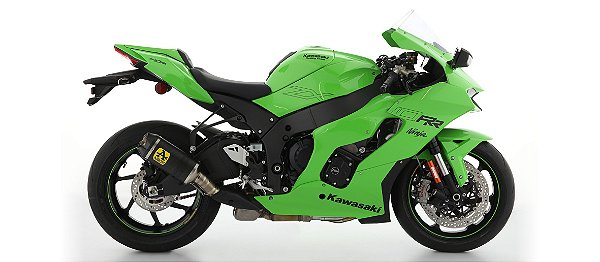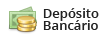# Ponteira Arrow Indy Race Kawasaki ZX10 R / ZX10 RR 21'~

Código: 71928 Marca:
Selecione a opção de Ponteira :
6x de R\$ 945,00
ou R\$ 5.216,40 via Depósito
Comprar Estoque: 60 dias úteis
6x de R\$ 945,00
ou R\$ 5.216,40 via Depósito
Comprar Estoque: 60 dias úteis
6x de R\$ 1.126,66
ou R\$ 6.219,20 via Depósito
Comprar Estoque: 5 dias úteis
6x de R\$ 1.126,66
ou R\$ 6.219,20 via Depósito
Comprar Estoque: 5 dias úteis
6x de R\$ 945,00
A partir de R\$ 5.670,00
ou R\$ 5.216,40 via Depósito
• 1x de R\$ 5.670,00 sem juros
• 2x de R\$ 2.835,00 sem juros
• 3x de R\$ 1.890,00 sem juros
• 4x de R\$ 1.417,50 sem juros
• 5x de R\$ 1.134,00 sem juros
• 6x de R\$ 945,00 sem juros
• 1x de R\$ 5.670,00 sem juros
• 2x de R\$ 2.835,00 sem juros
• 3x de R\$ 1.890,00 sem juros
• 4x de R\$ 1.525,01
• 5x de R\$ 1.237,71
• 6x de R\$ 1.046,32
• 7x de R\$ 909,72
• 8x de R\$ 807,38
• 9x de R\$ 727,88
• 10x de R\$ 664,35
• 11x de R\$ 612,45
• 12x de R\$ 569,28
• R\$ 5.216,40• 1x de R\$ 5.670,00 sem juros
• 2x de R\$ 2.835,00 sem juros
• 3x de R\$ 1.890,00 sem juros
• 4x de R\$ 1.417,50 sem juros
• 5x de R\$ 1.134,00 sem juros
• 6x de R\$ 945,00 sem juros
• 1x de R\$ 5.670,00 sem juros
• 2x de R\$ 2.835,00 sem juros
• 3x de R\$ 1.890,00 sem juros
• 4x de R\$ 1.525,01
• 5x de R\$ 1.237,71
• 6x de R\$ 1.046,32
• 7x de R\$ 909,72
• 8x de R\$ 807,38
• 9x de R\$ 727,88
• 10x de R\$ 664,35
• 11x de R\$ 612,45
• 12x de R\$ 569,28
• R\$ 5.216,40• 1x de R\$ 6.760,00 sem juros
• 2x de R\$ 3.380,00 sem juros
• 3x de R\$ 2.253,33 sem juros
• 4x de R\$ 1.690,00 sem juros
• 5x de R\$ 1.352,00 sem juros
• 6x de R\$ 1.126,66 sem juros
• 1x de R\$ 6.760,00 sem juros
• 2x de R\$ 3.380,00 sem juros
• 3x de R\$ 2.253,33 sem juros
• 4x de R\$ 1.818,18
• 5x de R\$ 1.475,65
• 6x de R\$ 1.247,46
• 7x de R\$ 1.084,61
• 8x de R\$ 962,59
• 9x de R\$ 867,80
• 10x de R\$ 792,07
• 11x de R\$ 730,19
• 12x de R\$ 678,71
• R\$ 6.219,20• 1x de R\$ 6.760,00 sem juros
• 2x de R\$ 3.380,00 sem juros
• 3x de R\$ 2.253,33 sem juros
• 4x de R\$ 1.690,00 sem juros
• 5x de R\$ 1.352,00 sem juros
• 6x de R\$ 1.126,66 sem juros
• 1x de R\$ 6.760,00 sem juros
• 2x de R\$ 3.380,00 sem juros
• 3x de R\$ 2.253,33 sem juros
• 4x de R\$ 1.818,18
• 5x de R\$ 1.475,65
• 6x de R\$ 1.247,46
• 7x de R\$ 1.084,61
• 8x de R\$ 962,59
• 9x de R\$ 867,80
• 10x de R\$ 792,07
• 11x de R\$ 730,19
• 12x de R\$ 678,71
• R\$ 6.219,20• 1x de R\$ 5.670,00 sem juros
• 2x de R\$ 2.835,00 sem juros
• 3x de R\$ 1.890,00 sem juros
• 4x de R\$ 1.417,50 sem juros
• 5x de R\$ 1.134,00 sem juros
• 6x de R\$ 945,00 sem juros
• 1x de R\$ 5.670,00 sem juros
• 2x de R\$ 2.835,00 sem juros
• 3x de R\$ 1.890,00 sem juros
• 4x de R\$ 1.525,01
• 5x de R\$ 1.237,71
• 6x de R\$ 1.046,32
• 7x de R\$ 909,72
• 8x de R\$ 807,38
• 9x de R\$ 727,88
• 10x de R\$ 664,35
• 11x de R\$ 612,45
• 12x de R\$ 569,28
• R\$ 5.216,40* Este prazo de entrega está considerando a disponibilidade do produto + prazo de entrega.